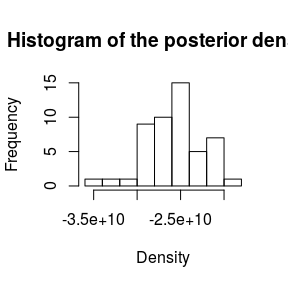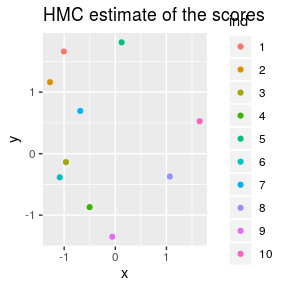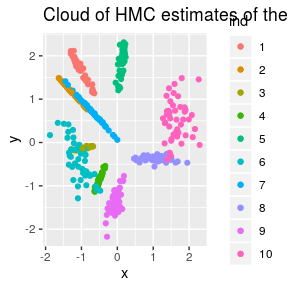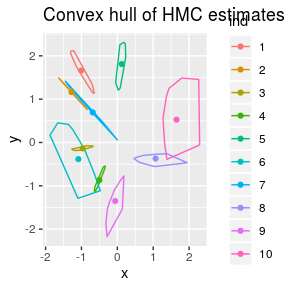# DrBats Model Evaluation

## Overview

This document is part of the “DrBats” project whose goal is to implement exploratory statistical analysis on large sets of data with uncertainty. The idea is to visualize the results of the analysis in a way that explicitely illustrates the uncertainty in the data.

The “DrBats” project applies a Bayesian Latent Factor Model.

This project involves the following persons, listed in alphabetical order :

• Bénédicte Fontez (aut)

• Nadine Hilgert (aut)

• Susan Holmes (aut)

• Gabrielle Weinrott (cre, aut)

require(DrBats)
mycol<-c("#ee204d", "#1f75fe", "#1cac78", "#ff7538", "#b4674d", "#926eae",
"#fce883", "#000000", "#78dbe2", "#6e5160", "#ff43a4")

data("toydata")
data("stanfit")

We pick up where the last vignette modelFit.pdf leaves off, with a post-processed mcmc.list object called codafit.

codafit <- coda.obj(stanfit)

In order to evaluate the model and visualize the results, we calculate the posterior density with the $$postdens()$$ function. We can draw a histogram for this posterior (un-normalized) density, once we have specified the original data, the number of latent factors, the chain we want to look at :

post <- postdens(codafit, Y = toydata$Y.simul$Y, D = toydata$wlu$D, chain = 1)
hist(post, main = "Histogram of the posterior density", xlab = "Density")The following plot shows the $$10$$ MCMC estimates for the coordinates of each observation.

beta.res <- visbeta(codafit, toydata$Y.simul$Y, toydata$wlu$D, chain = 1, axes = c(1, 2), quant = c(0.05, 0.95))

ggplot2::ggplot(beta.res$mean.df, ggplot2::aes(x = x, y = y, colour = ind)) + ggplot2::geom_point(ggplot2::aes(x = x, y = y, colour = ind)) + ggplot2::ggtitle("HMC estimate of the scores")Other possibilites are available to better visualize the uncertainty of the estimate. You can choose to plot all of the realizations of the MCMC chain at a certain level of confidence defined by the parameter $$quant$$: ggplot2::ggplot() + ggplot2::geom_point(data = beta.res$points.df, ggplot2::aes(x = x, y = y, colour = ind)) +
ggplot2::geom_point(data = beta.res$mean.df, ggplot2::aes(x = x, y = y, colour = ind)) + ggplot2::ggtitle("Cloud of HMC estimates of the scores")But that’s a bit messy, so we also propose the convex hull at, for instance, 95% for the estimate. ggplot2::ggplot() + ggplot2::geom_path(data = beta.res$contour.df, ggplot2::aes(x = x, y = y, colour = ind)) +
ggplot2::geom_point(data = beta.res\$mean.df, ggplot2::aes(x = x, y = y, colour = ind)) +
ggplot2::ggtitle("Convex hull of HMC estimates of the scores")